# The BriefA Blog about the LSAT, Law School and Beyond

The name “triangle” means, sensibly enough, “three angles” and indeed every triangle has three angles and three sides: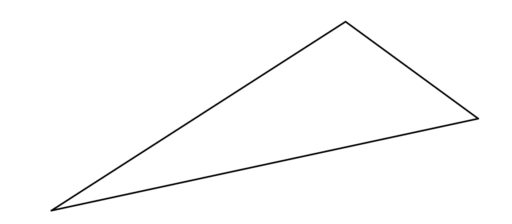This much is hopefully familiar. But just as with circles, there are many special terms people have devised for triangles. When it comes to the sides of a triangle, we can have:

Equilateral Triangles: All sides have the same length.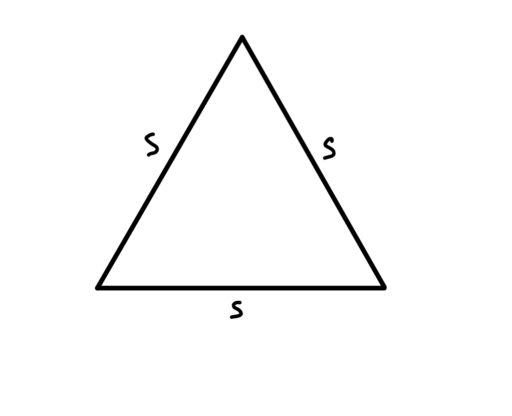Isosceles Triangles: Two sides have the same length as each other; the third has a different length.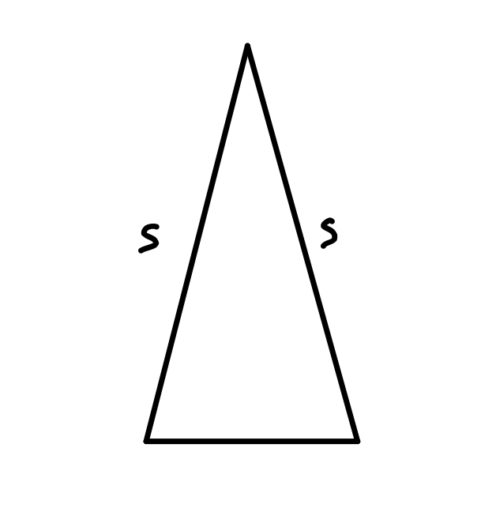Note that in an isosceles triangle:

Equal Sides Have Equal Angles: In an isosceles triangle, the angles opposite from the sides of equal length must have equal degree.

Thus, in the below diagram: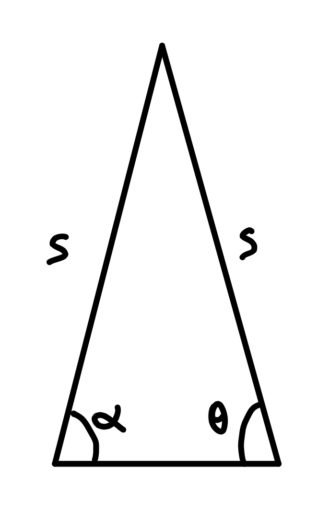anglesandare equal.

Scalene Triangles: All of the sides are of different lengths.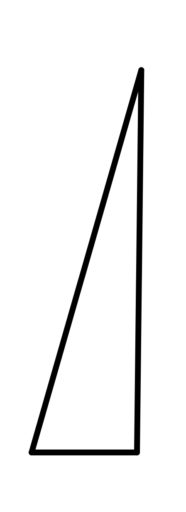We can also classify triangles according to their angles:

Acute Triangles: All angles are less than 90 degrees.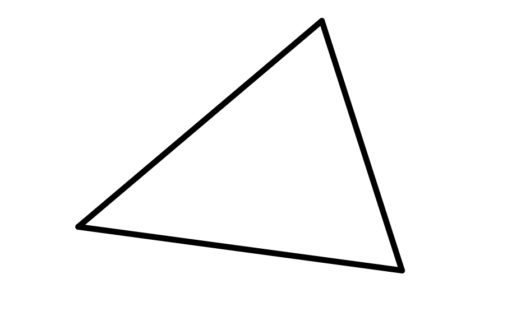Right Triangles: One angle is exactly 90 degrees.

We use a square to mark a 90 degree angle.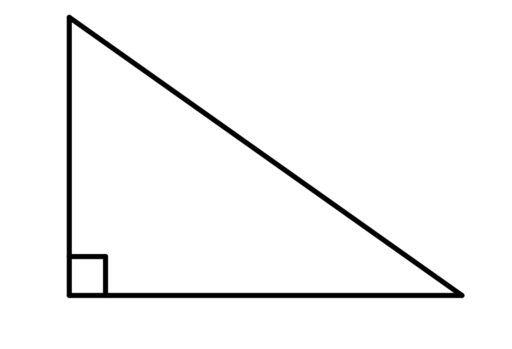Right triangles have lots of special further features which we will talk about here.

Obtuse Triangles: One angle is more than 90 degrees.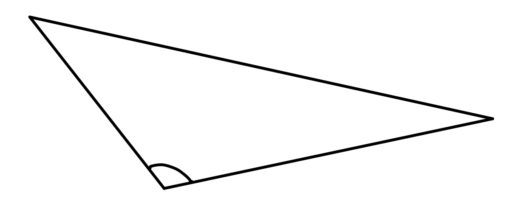Now, we can also compare two triangles to each other. We can say that they are:

Similar: Two triangles are similar if their angles have the same values.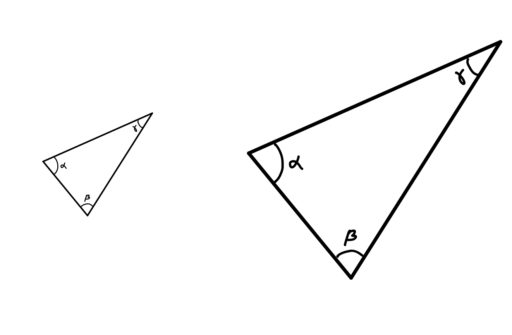So in the above diagram, even though one of the triangles is obviously bigger than the other, we can say that they are “similar” (in the technical, mathematical sense defined above) because their angles have the same values. And the definition makes sense since, after all, those two triangles do look pretty similar (in our ordinary, day-to-day sense)!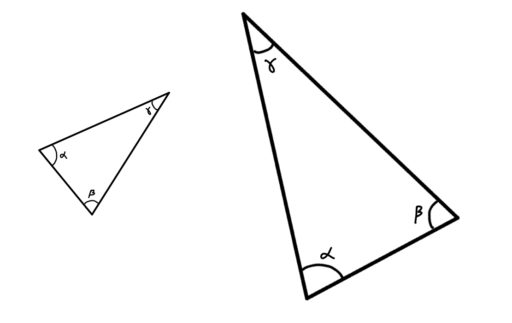These triangles are also similar to one another; it doesn’t matter if you rotate or flip the second triangle. All that matters is whether the angle values for the two figures are the same.

The reason why we care about the similarity of triangles is:

Similar Triangles Share Proportions: If two triangles are similar, then there is some constant ratio you can multiply the sides of one triangle by in order to get the sides of the other triangle.

For example, if the following two triangles are similar: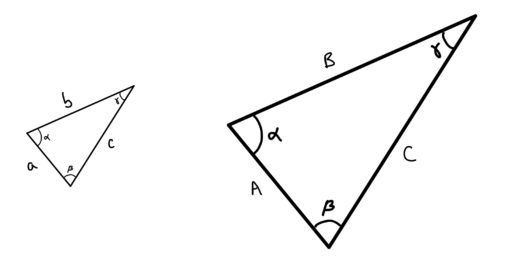then there is some constant, call it, such that:This can be very helpful in trying to find certain side lengths or the area of a triangle as we shall see.

Congruent: Two triangles are congruent if their angles are the same and their sides are the same length.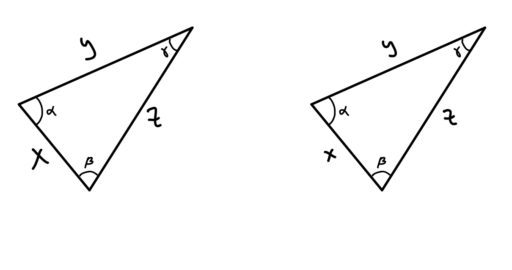You can think of congruence as signaling that those two triangles are effectively the same triangle. The lines are the same length, the angles are the same, the area is the same, and so on.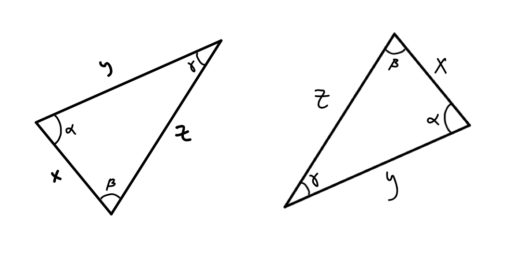And again, it doesn’t matter if you rotate or flip one of the triangles. It is still true that their angles and side lengths are the same, since rotating or flipping a shape won’t change any of that.

Now, when it comes to finding the area of a triangle, you need to know the base of the triangle and its height. The base can be any side of the triangle, but the height needs to extend perpendicular (at a right angle to) the base, and reach the highest point of the triangle, away from the base, like so: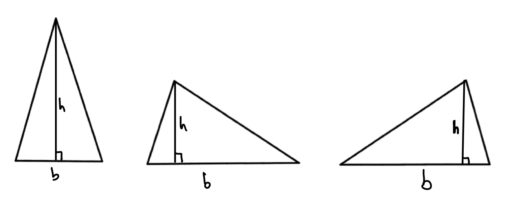Traditionally, we useto denote the base andto denote the height. Thus, we get:

Triangle Area Formula:Finally, we note two fundamental facts about triangles:

Angles of a Triangle Sum to 180: For any triangle, the sum of its interior angles is 180 degrees.

Triangle Inequality: The sum of the length of any two sides of a triangle is greater than the length of its third side.

Practice Problems:

1. Find the value of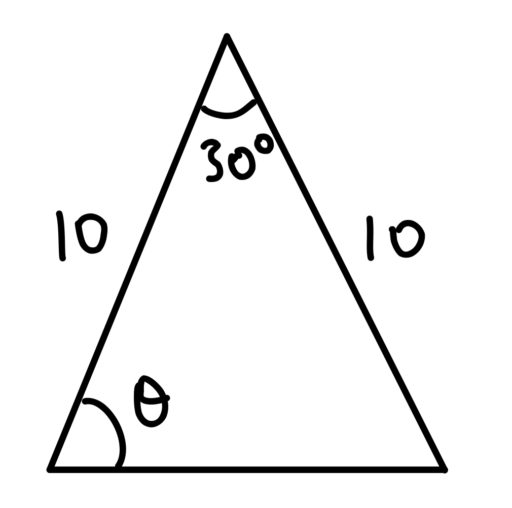2. Find the value of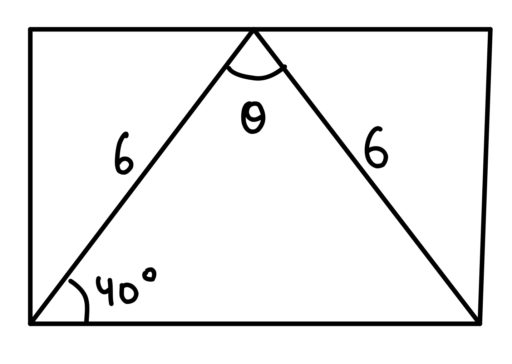3. A triangle has legs that areandunits long. What integer values are possible for the third side?

Comment on this

No doubt we’re all familiar with circles: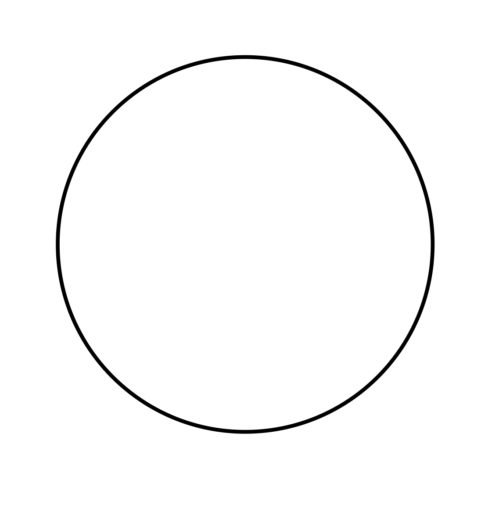Less familiar, though, may be the many technical terms for certain parts of the circle — the kinds of terms that most people leave behind in high school. But knowing what those terms mean can help you on the GRE by giving you a vocabulary that helps you to understand the formulas and the problems (it’s easier to learn how to find the area of a circle’s sector, rather than “the bit of the circle left over when you cut off the circular bit”).

Circle: A 2D shape whose points are always the same distance from some central point.

Center: The center of a circle is the point within the circle from which every point of the circle is the same distance. We often label the center and use it to identify the circle (e.g. “the circle whose center is at A”).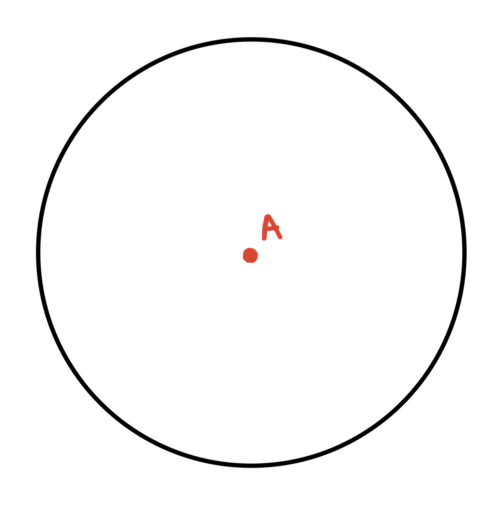Radius: Any line from the center of a circle to any point on the circle. We often use the variablefor the length of the radius.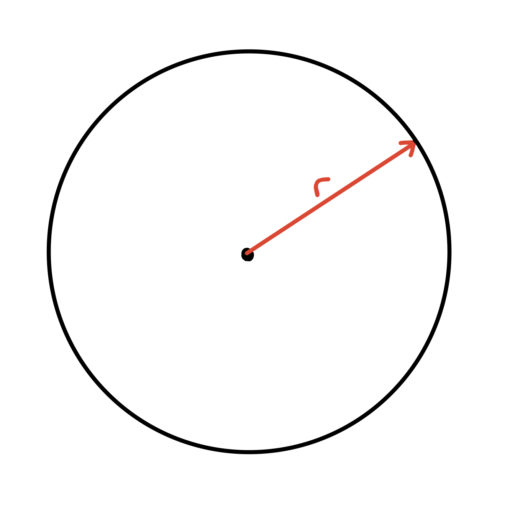The radius of a circle is often very important because it allows us to find the area of that circle:

Area of a Circle: The area of a circle with radiusisDiameter: Any line that connects two points on the circle and the center of the circle. We often use the variablefor the length of the diameter.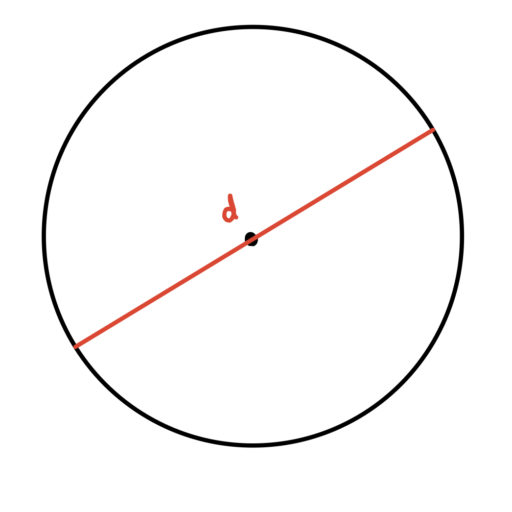And of course, using the diameter, we can always find the radius of a circle:

Diameter Formula: For a circle with radius, the diameterCircumference: The distance around the circle.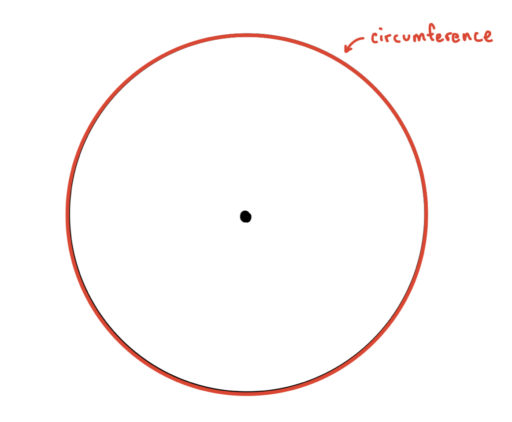And we can find the circumference of a circle using the diameter:

Circumference Formula: The circumference of a circle with a radiusisThus, the area of a circle, its radius, its diameter, and its circumference are all related. Using any one of these values, we can find the other three!

Chord: Any line segment that connects two points on a circle.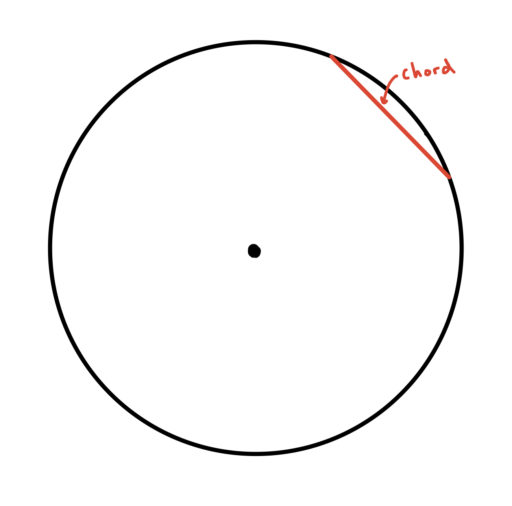Note also that:

Diameter is Longest Chord: The diameter of a circle is also a chord of the circle and, in fact, it is the longest chord on a circle.

Arc: Any portion of a circle’s circumference located between two points on the circle.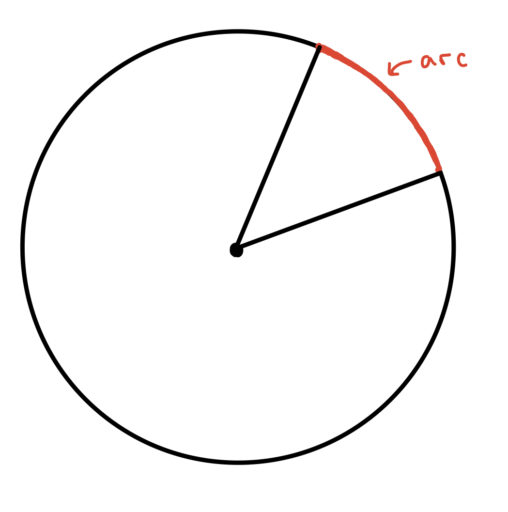Sector: A portion of the circle enclosed by two radii and an arc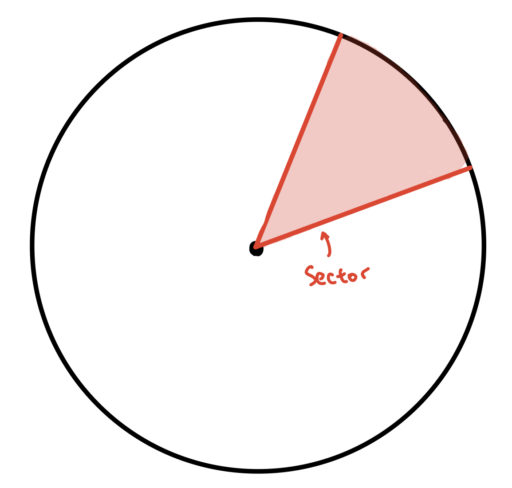Some GRE problems will ask you to find the area of some sector of the circle. We will talk about how to handle those problems later (%TODO insert hyperlink here).

Tangent: A straight line that touches a curve or circle at a single point

Point of Tangency: The point at which a tangent line touches a curve. Note that the tangent line (in red below) will be perpendicular to any line connecting the center and the point of tangency.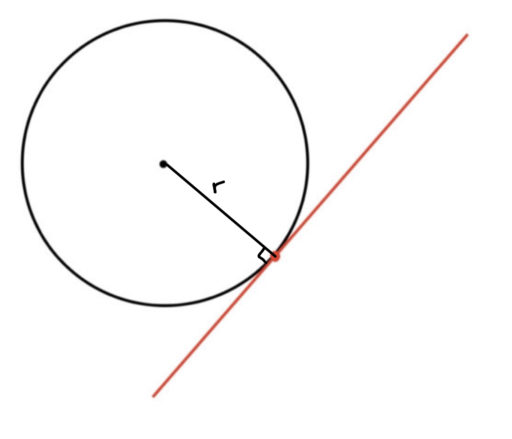Finally, circles can be drawn within or around other shapes:

Inscribed: Circles can be inscribed within other shapes. An inscribed circle is the largest possible circle that can be drawn wholly within another shape. For example: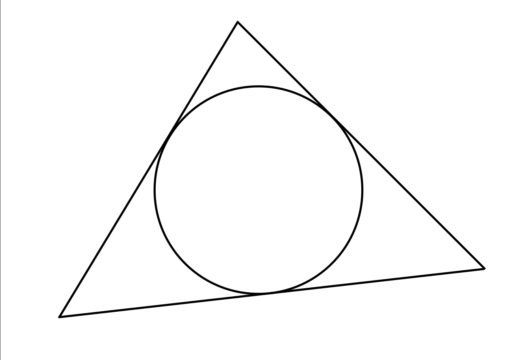Circumscribed: Circles can also be drawn around other shapes. A circumscribed circle is the smallest possible circle that can be drawn wholly outside of another shape. For example: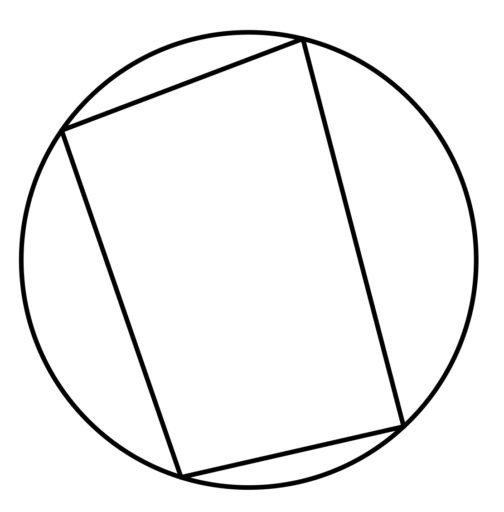And circles can share a center:

Concentric Circles: Two circles are concentric if they share the same center.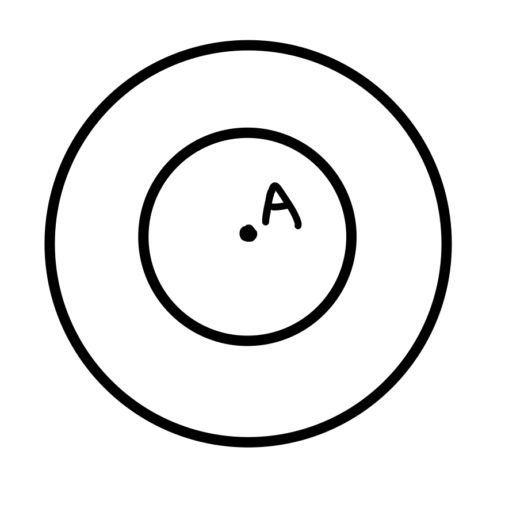Practice Problems:

1. Draw a circle inscribed within a square

3. The radius of the below circle is 1. What is the area of the shaded region?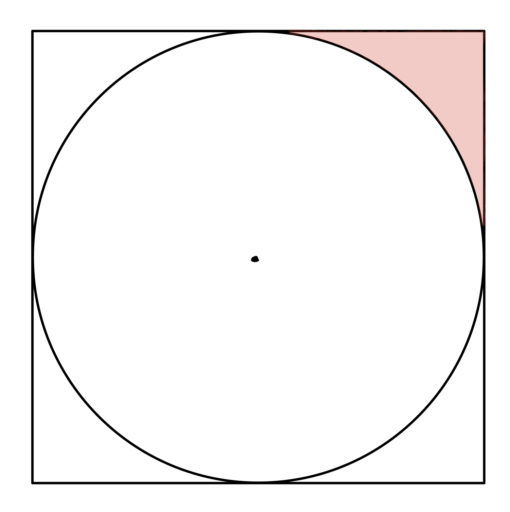4. Which is longer?A.is longer.
B.is longer.
C. They are equally long.
D. It cannot be determined.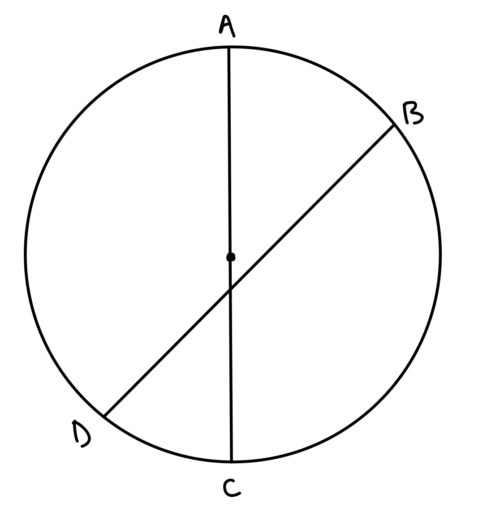5. The radius of the following inscribed circle is 5, and the radius drawn connects to a point of tangency. What is the area of the shaded region?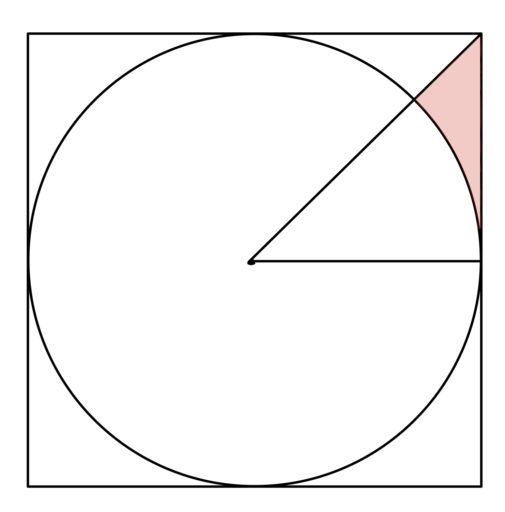6. The shaded region has an area of 5. What is the area of the circle?Comment on this

Many GRE math problems involve 2D shapes, which include triangles, squares, and in general, the kind of shapes you might remember seeing in first grade. It turns out these shapes actually have a number of interesting properties, knowledge of which matters for doing well on the GRE. These shapes are often defined by the number of sides they have. Now, there is no 2D shape that is made of just 1 line: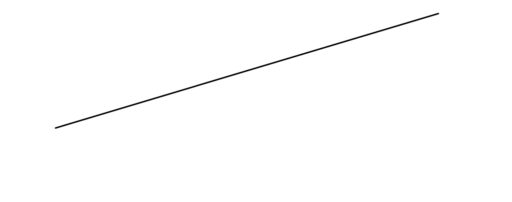And similarly, there is no 2D shape with just 2 lines: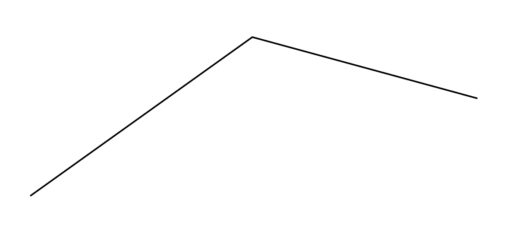But starting with three lines, we get:No doubt you know that this is called a triangle. With four lines we get the general category of quadrilaterals (or four-sided shapes):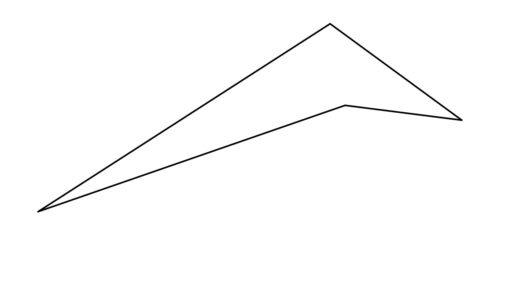This category includes trapezoids, squares, rectangles, parallelograms, and rhombuses: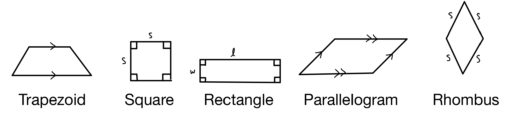(The arrows above indicate that those two lines are parallel to one another).

And we can also have a 2D shape defined by a constant distance from a central point: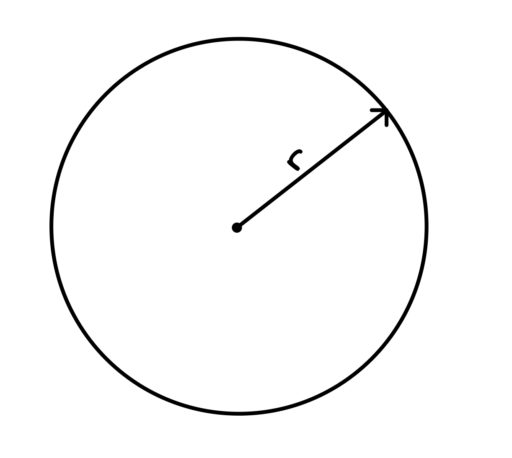and we call this a circle.

Now, of courses there are other 2D shapes; we can have pentagons (5 sides), hexagons (6 sides), and more. (A chiliagon has 1000 sides). But for the GRE, we will generally need just the above.

Finally, we also need the idea of a regular polygon.

Regular Polygon: Regular polygons have sides of equal length and angles of equal degree.

So, for example, the regular 3-sided polygon is the equilateral triangle: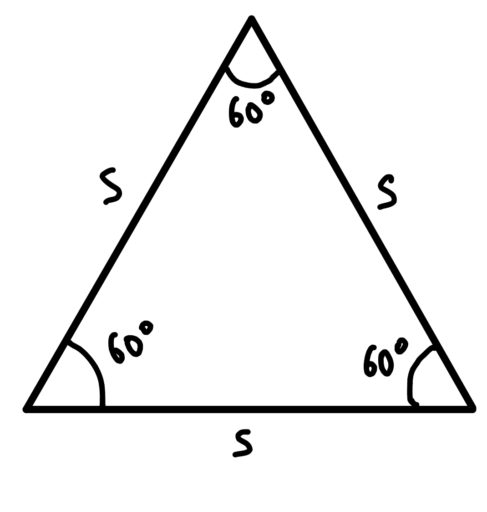which, as the name equi-lateral suggests, has three sides of equal length. And its angles are all 60 degrees.

The regular polygon for four sides is the square: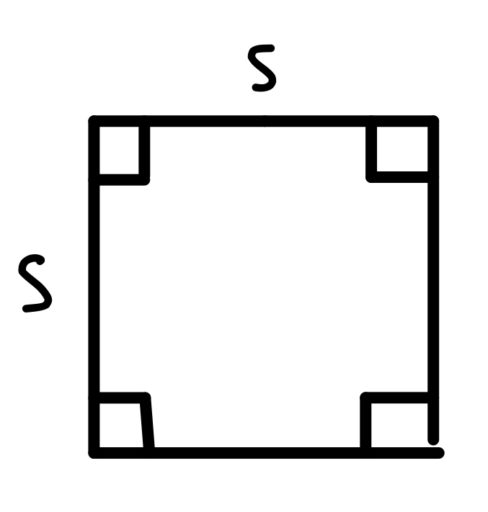where each angle is 90 degrees.

You may have noticed a pattern here: 60 degrees for 3 sides; 90 degrees for 4 sides. And this pattern holds for any number of sides:

Angles of a Regular Polygon
If we have a regular polygon ofsides, each angle isdegrees.

This formula can be important when trying to find certain angle values; for example:

Example 1
You have a seven-sided regular polygon. Give the degree of an angle of the polygon.

Example 2
You have a regular octagon. What is the sum of all of the angles of the octagon?

And the fact that regular polygons have sides of equal length can help in problems involving the perimeter of a regular polygon:

Example 3
A regular 29-sided polygon has a perimeter of 116. What is the length of its sides?

Comment on this

Geometry, unlike the other mathematical subjects tested on the GRE, has special rules. Basically, these rules prohibit test-takers from just eye-balling the answers based off of the diagrams. So for example, in the following problem:

Example 1
Find the value of: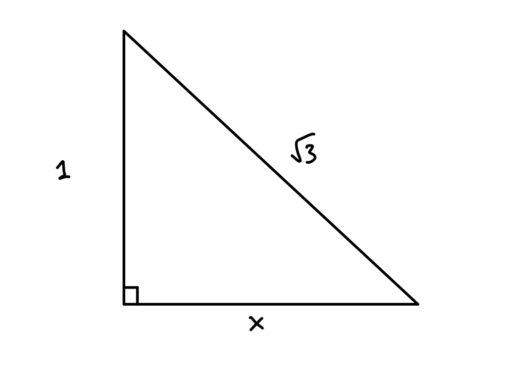You cannot just look at the figure and guess that the mystery leg is the same length as the other leg of the triangle. For if we used the Pythagorean Theorem to calculate the solution:

So our first rule is:

Rule 1: Shapes and figures are not necessarily drawn to scale.

So in the following problem:

Example 2
Is side B longer than side A?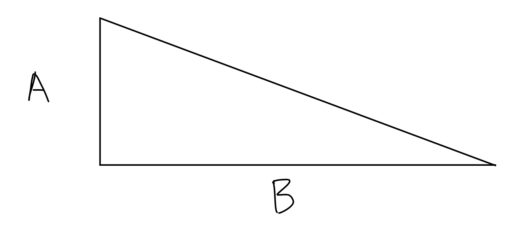The correct answer is that it cannot be determined which side is longer.

However, this does not mean that the figures drawn are totally misleading. For we also have:

Rule 2: Shapes and figures do show the relative position of different objects.

What does this mean? It means that if you see something like: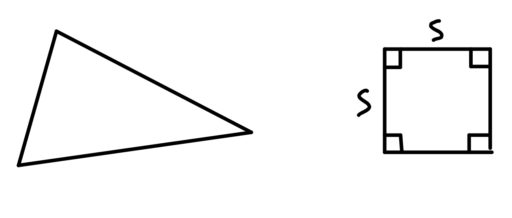Then you can conclude that the triangle is to the left of the square. Or if you see: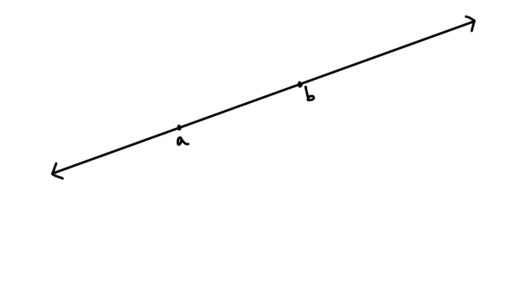You can conclude that those two points are on that line, and that point a is to the left of point b.

Finally, the rules are different for co-ordinate systems and the number line:

Rule 3: Co-ordinate systems and the number line are to scale.

The main co-ordinate system you will see on the GRE is the xy-plane: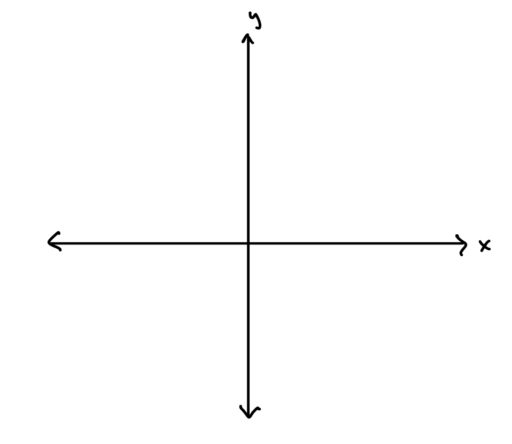and the number line is just the following diagram: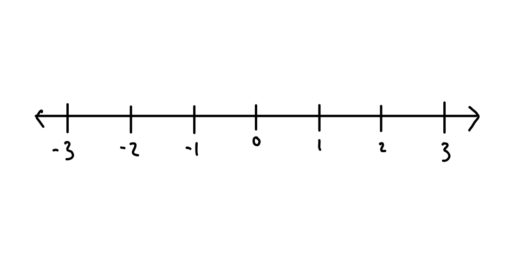On such systems, the objects are to scale. So if you see: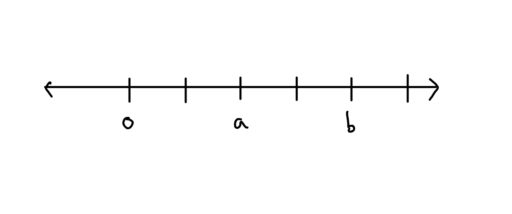you can conclude that the value foris twice as large as the value forOr if you see: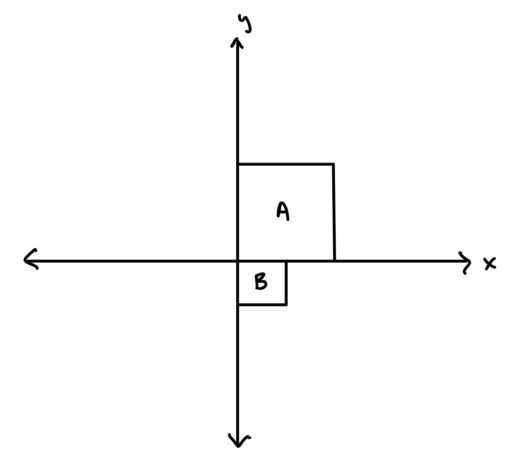you can conclude that the area of the smaller square is 1/4 of the area of the larger square.

So in short, remember that the proportions of shapes cannot be trusted, but the relative position of shapes can be trusted, and if there is co-ordinate system or a number line, then even the proportions can be trusted.

Comment on this

This guide covers all the geometry you need for the GRE math section. We will try to make the content fairly intuitive, combining formulas with some explanation of why the formula makes sense. Doing well on geometry problems can make a big difference for your GRE math score since geometry questions make up about 15% of all GRE math questions.

Most geometry questions will ask you to do one of four things:

1. Find the line length
Example 1
In the following figure, find the value of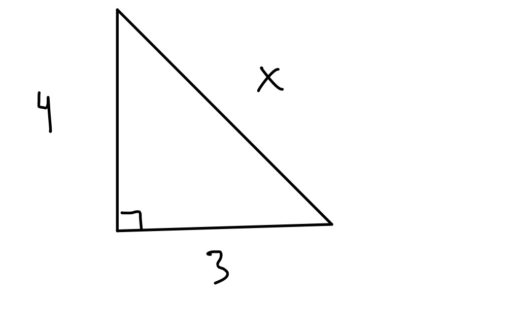2. Find the angle
Example 2
Find the value of: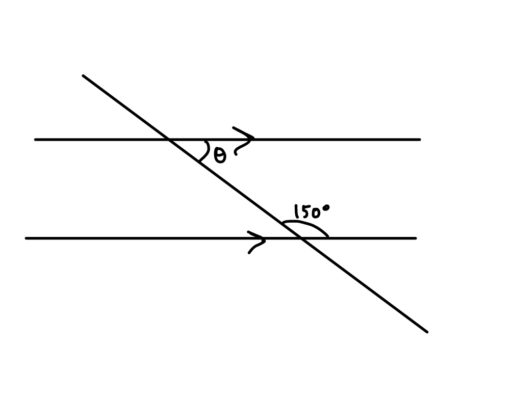3. Find the area (of a 2D shape) or surface area (of a 3D shape), or
Example 3
Find the area of the shaded region: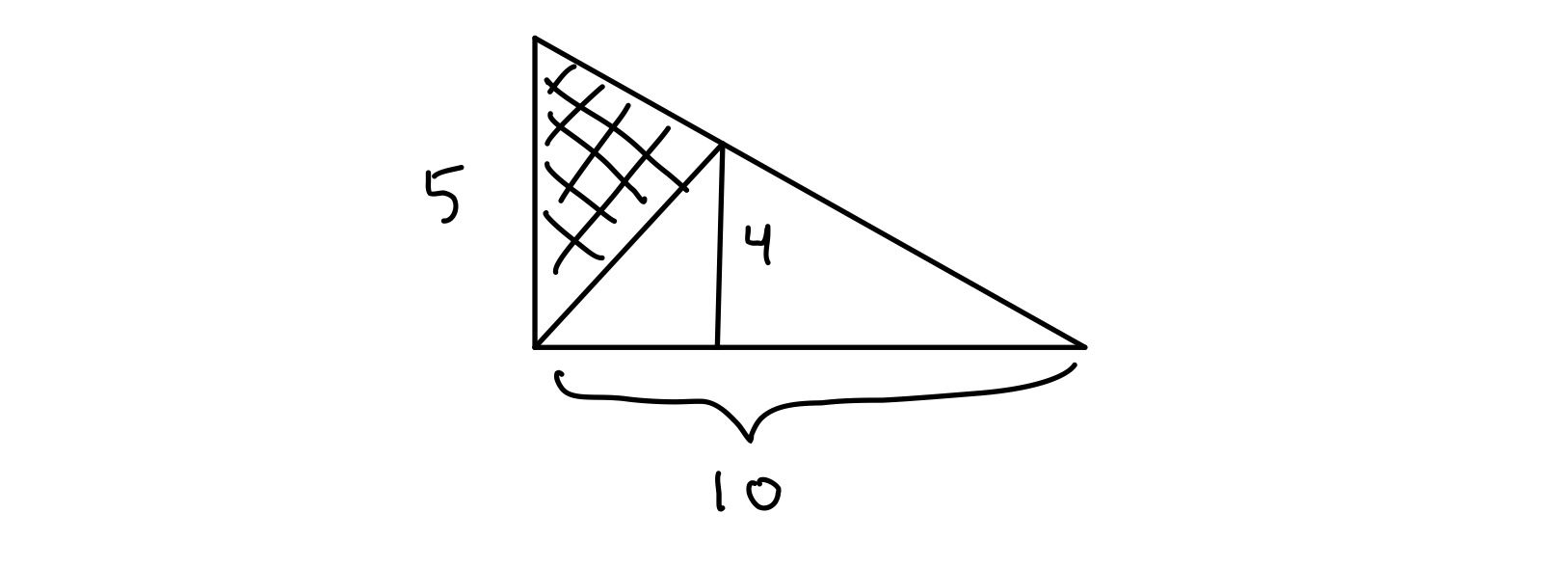4. Find the volume (of a 3D shape)
Example 4
Find the volume of the rectangular prism below: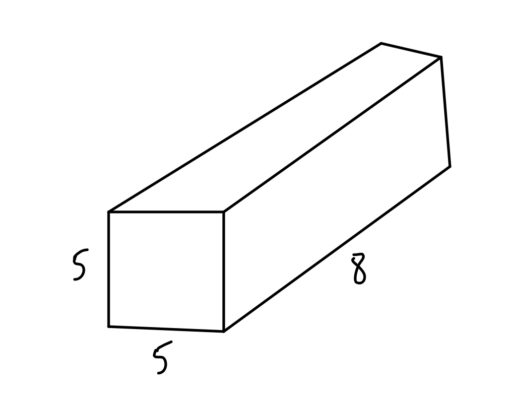But these problems can be difficult because they give you seemingly odd bits of information. For example, they might ask:

Example 5
The area of the following triangle is 20. Find: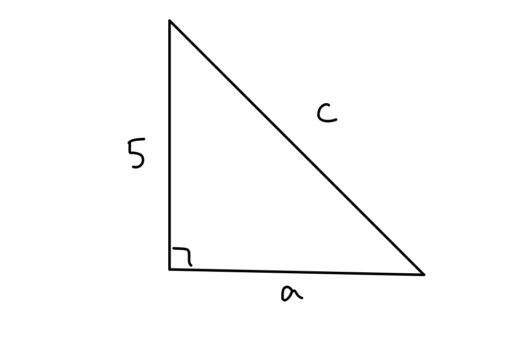and you will have to figure out a way to use what you know (the area formula for triangles, the Pythagorean Theorem) to figure out the answer:

There are many little factoids which matter in geometry, and these will be found throughout our guide. Now, the sheer quantity of formulae can get overwhelming, so we will distinguish between must-know formulas like:

Pythagorean Theorem: For any right triangle whereare the legs of the triangle andis the hypotenuse,and the less critical formulas like:

30-60-90 Right Triangle: For any right triangle with angles of 30, 60, and 90 degrees, the side lengths have the following ratio: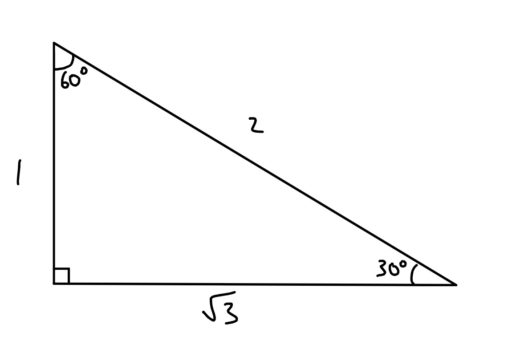The must-know formulas are, well, a must-know if you want to do well on geometry. But if you are okay with missing a few geometry questions on the exam, you can afford to ignore some of the less critical formulas. Each such formula comes up maybe once per test.

Comment on this

Welcome to Law School Success Stories, where we discuss 7Sage applicants who made the most of their GPA and LSAT score.

👤 Who: “Sanjay,” a recent college grad

• 📈 Top LSAT: 170
• 📉 GPA: 2.99

Results

• 🏆 Accepted at UVA

🔎 Initial Assessment

Sanjay had applied the previous cycle and been accepted to GW and Fordham with substantial scholarships. During the year since applying, he had taken the LSAT two more times (for a total of five attempts), ultimately raising his score from 155 to a whopping 170. This made him determined to take another crack at the T-14, despite having a GPA well below their 25th percentiles.

A phone call with Sanjay revealed that he was not only a finance whiz, but a funny and down-to-earth guy. His low GPA had been due, in large part, to a debilitating skin condition that struck him at the beginning of college. The resulting social anxiety caused him to miss classes frequently during his first and second years. Sanjay saved up enough money to begin a new treatment regimen, and by the fall of his third year, he was earning good grades.

Our task, then, was to convince the admissions committee at a T-14 school that his GPA didn’t reflect his ability to succeed in law school, and that Sanjay was so smart, ambitious, and congenial that they simply could not turn him down. We developed a three-part strategy:

Comment on this

Once upon a time, the LSAT was the only game in town for law school applicants. Things began to change in 2016, when the University of Arizona Law allowed applicants to apply with a GRE score, followed by Harvard Law the next year. Nearly forty law schools now accept the test.

## The Case for Taking the GRE

The GRE has a lot of advantages from the perspective of a test-taker. Available throughout the year and across the world, it’s easier to schedule and more convenient than the LSAT. The GRE will also feel more familiar to anyone who’s taken the SAT or ACT, and most test-takers find it less time-pressured than the LSAT. The test has special advantages for applicants with quantitative skills, who may find it easier than the LSAT, and for anyone applying to dual-degree programs, who may have to take the GRE in any case. Finally, if an applicant decides to cancel her GRE score, the test doesn’t show up on her record. If an LSAT-taker cancels her score, by contrast, the test still shows up on her score report.

Nevertheless, there are some reasons to hesitate before you go all in on the GRE.

Featured image: Person Behind Books by Pixabay via Pexels is free to use without attribution.